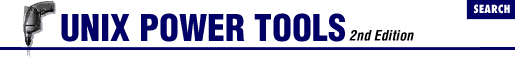home | O'Reilly's CD bookshelfs | FreeBSD | Linux | Cisco | Cisco Exam

#Chapter 47C Shell Programming...NOT## 47.3 Conditional Statements with if

.login and .cshrc files" 1070 is the use of conditionals (if statements). This article explains the syntax of if statements. Article 47.4 of explains the syntax of the expressions you can test with an if .

The if command is used to begin a conditional statement. The simple format is:

```if (```expr
```
) ```cmd
```
```

There are three other possible formats, shown side-by-side:

```if (```expr
```
) then   if (```expr
```
) then   if (```expr
```
) then
```cmds
```
```cmds1
```
```cmds1
```

endif   else   else if (```expr
```
) then
```cmds2
```
```cmds2
```

endif   else
```cmds3
```

endif```

In the simplest form, execute ```cmd ``` if ```expr ``` is true; otherwise do nothing (redirection still occurs; this is a bug). In the other forms, execute one or more commands. If ```expr ``` is true, continue with the commands after `then` ; if ```expr ``` is false, branch to the commands after `else` (or after the `else if` and continue checking). For example, the following if clause will take a default action if no command-line arguments are given:

```if (\$#argv == 0) then
echo "No filename given. Sending to Report."
set outfile = Report
else
set outfile = \$argv
endif```

For more examples, see article 47.4 .

- DG from O'Reilly & Associates' UNIX in a Nutshell (SVR4/Solaris)47.2 C Shell Programming Considered Harmful47.4 C Shell Variable Operators and Expressions